# RF-MOVE Surfaces Version 5

Online manuals, introductory examples, tutorials, and other documentation.

## RF-MOVE Surfaces Version 5

This module window manages the load parameters of the movable loads. You can combine different loads in one Load Model moving as a "load block" over the surface.

A set of moving loads includes one or several loads describing the moving load by forces and moments. The number of the first set of moving loads is preset. You can overwrite the description Load model 1 manually.

If moving loads on surfaces are running parallel or with an offset, another set of moving loads should be created with the [New] button. Thus, an individual control of moving loads within one set of movements is possible (see chapter 2.4).

The buttons have the following functions:

Table 2.2 Buttons for managing sets of moving loads
Button Function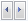Goes to next or previous set of moving loads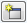Creates a set of moving loads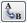Allows for renumbering a set of moving loads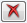Deletes current set of moving loads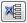Deletes all sets of moving loads without any warning

The column titles are synchronized to the selected load type and distribution.

Type

A load can be defined as Force or Moment. You can access the list with the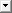button or the function key [F7].

The list offers different options to represent the load's effect. The selected load is shown symbolically in the graphic below the table column.

Concentrated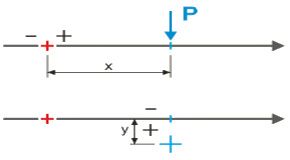Single force, single moment
In addition to the load magnitude, enter the load's distances x along and y at right angle to the fixed point of the load position.

Line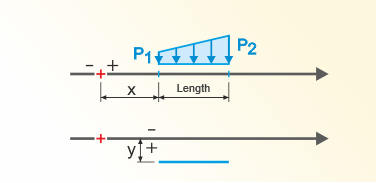Enter the forces P1 and P2, the distances x and y as well as the Length of the line load.

Rectangular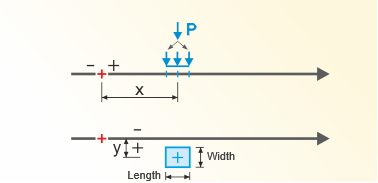In addition to the distances x and y, define the load magnitude P. It is converted into an area load acting on the Width and Length to be specified.

Circular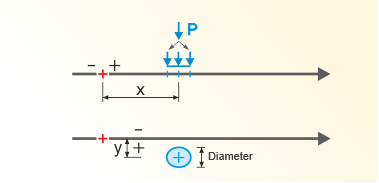In addition to the distances x and y, define the load magnitude P. It is converted into a circular area load acting on the Diameter to be specified.

Axle - Concentrated Forces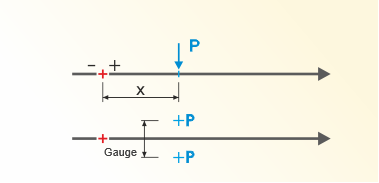Load P is distributed on two single loads in a distance of the Gauge. Enter also the distance x to the fixed point of the load position.

Axle - Rectangular Areas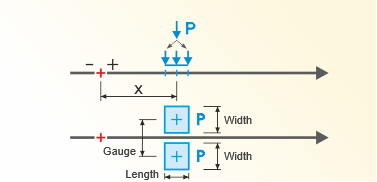The load P is distributed on two area loads in a distance of the Gauge. They each act on the Width and Length to be specified (e.g. wheel contact area).

Axle - Circle Areas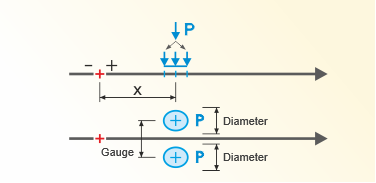The load P is distributed on two area loads in a distance of the Gauge. They each act on a circular area with the Diameter to be specified.

Left - Endless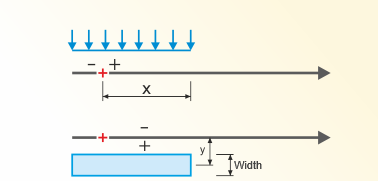At each load position, a constant load is applied which is effective to the point where the set of lines starts. In addition to the load magnitude, enter the Width and the distance of the right load edge to the load step's fixed point.

Right - Endless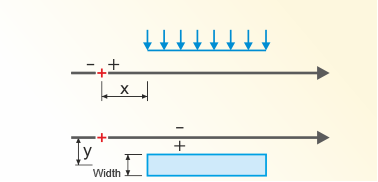At each load position, a constant load is applied which is effective to the point where the set of lines ends. In this case, enter the distance of the left load edge to the fixed point.

Whole Length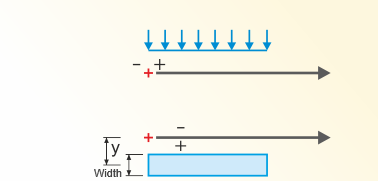A constant load acts on the total length of the set of lines. In addition to the load magnitude, enter the Width of the load. The load can be shifted, if necessary, in the distance y at right angle to the moving line.

Direction

The effect of loads can be defined Local in direction of the surface axes, or Global in direction of the axes X, Y and Z. You can access the list with thebutton or the function key [F7].

x

The location x describes the distance of the load to the load step's fixed point along the moving line. This point is symbolized by a red cross in the sketch.

A positive value moves the loads in the viewing direction of the set of lines. If x is negative, the load is placed before the center of the load step. This way, it is possible to model tandem axle loads entering the runway of surfaces.

y or Gauge

This column allows you to place loads alongside the moving line. A positive y-value arranges the load in viewing direction to the right of the moving line, a negative value to the left.

Here, loads can be individually shifted at right angle to the moving line. By contrast, the Eccentricity in window 1.2 Sets of Lines shifts all loads.

If axle loads are set, table column E gets the title Gauge. Then, the value describes the load pair's centroidal distance according to the sketch.

P or M

In this column, you can enter the magnitudes of the force P or the moment M.

For a line load you have to enter the magnitude of the load P1 at the line start. The load value P2 at the line end can then be defined in the next column. If both values differ from each other, a trapezoidal load is applied.

Width

For rectangular loads you have to define the load width according to the sketch. It represents the dimension of the load contact patch at right angle to the moving direction.

If an Endless load is applied, you have to specify the width of the lane on which the uniformly distributed load is acting.

Length

In this column, you have to specify the length of the load contact patch in the direction of the moving direction, respectively the length of the line load.

The geometric conditions are taken into account when using offsets or bumpers (see chapter 2.2): The areas only get proportional loads from the contact patches.

Comment

This input field allows for a user-defined description of load parameters.

Library

Combining movable loads usually involves a lot of effort. But many load models are stored in a database that you can access with the [Library] button.

Filter

Use the list in this window section to filter the load models by different criteria.

List of Models

In the Load type list, you can find sets of moving loads described in bridge construction standards . When activating a row in the table, the data record of the respective load model is shown in the Load Parameters section below.

The list of load models contains axle and surface loads representing the effects from truck transport and car traffic. It is being constantly expanded and adjusted to the updated standards.

If a dispersal layer is applied, you can specify its thickness t in the input field. By taking into account the Angle of dispersal α, this layer produces a distribution of the contact patch loads. The Width and Length load parameters will be adjusted accordingly in the window section below.

The thickness of the RFEM surface won't be automatically used for the load distribution.

In window 1.3, you can save the current set of moving loads so that it can be used in other projects. You can find the [Save] button below the Load Parameters table. It opens the New Model dialog box.

As soon as the Model and Description is entered, you can [Save] the new load model.